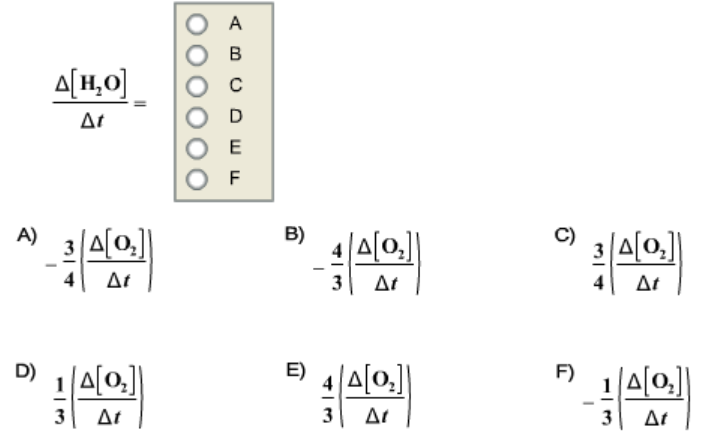# Problem: Given the following balanced chemical equation 3 O2+ 2 CH3OH → 2 CO2 + 4 H2O How is the rate of appearance of H2O related to the rate of disappearance of O2?

###### FREE Expert Solution

For this problem, we have to determine the rate of appearance of H2O related to the rate of disappearance of O2

The rate of a chemical reaction is the speed at which the reactants are converted into products.

It is expressed as the change in concentrations of reactants or products over time.

The given reaction is:

3 O2+ 2 CH3OH → 2 CO+ 4 H2

87% (184 ratings)###### Problem Details

Given the following balanced chemical equation

3 O2+ 2 CH3OH → 2 CO+ 4 H2

How is the rate of appearance of H2O related to the rate of disappearance of O2?Frequently Asked Questions

What scientific concept do you need to know in order to solve this problem?

Our tutors have indicated that to solve this problem you will need to apply the Average Rate of Reaction concept. You can view video lessons to learn Average Rate of Reaction. Or if you need more Average Rate of Reaction practice, you can also practice Average Rate of Reaction practice problems.